## Key Concepts

Review core concepts you need to learn to master this subject

### Grammar of Graphics with ggplot2

ggplot2 uses the basic units of the “grammar of graphics” to construct data visualizations in a layered approach.

The basic units in the “grammar of graphics” consist of:

• The data or the actual information that is to be visualized.
• The geometries, shortened to “geoms”, which describe the shapes that represent the data. These shapes can be dots on a scatter plot, bar charts on the graph, or a line to plot the data. Data are mapped to geoms.
• The aesthetics, or the visual attributes of the plot, including the scales on the axes, the color, the fill, and other attributes concerning appearance.

Visualizations in ggplot2 begin with a blank canvas, which is just an empty plot with data associated to it. Geoms are “added” as layers to the original canvas, adding representations of the data to the visualization.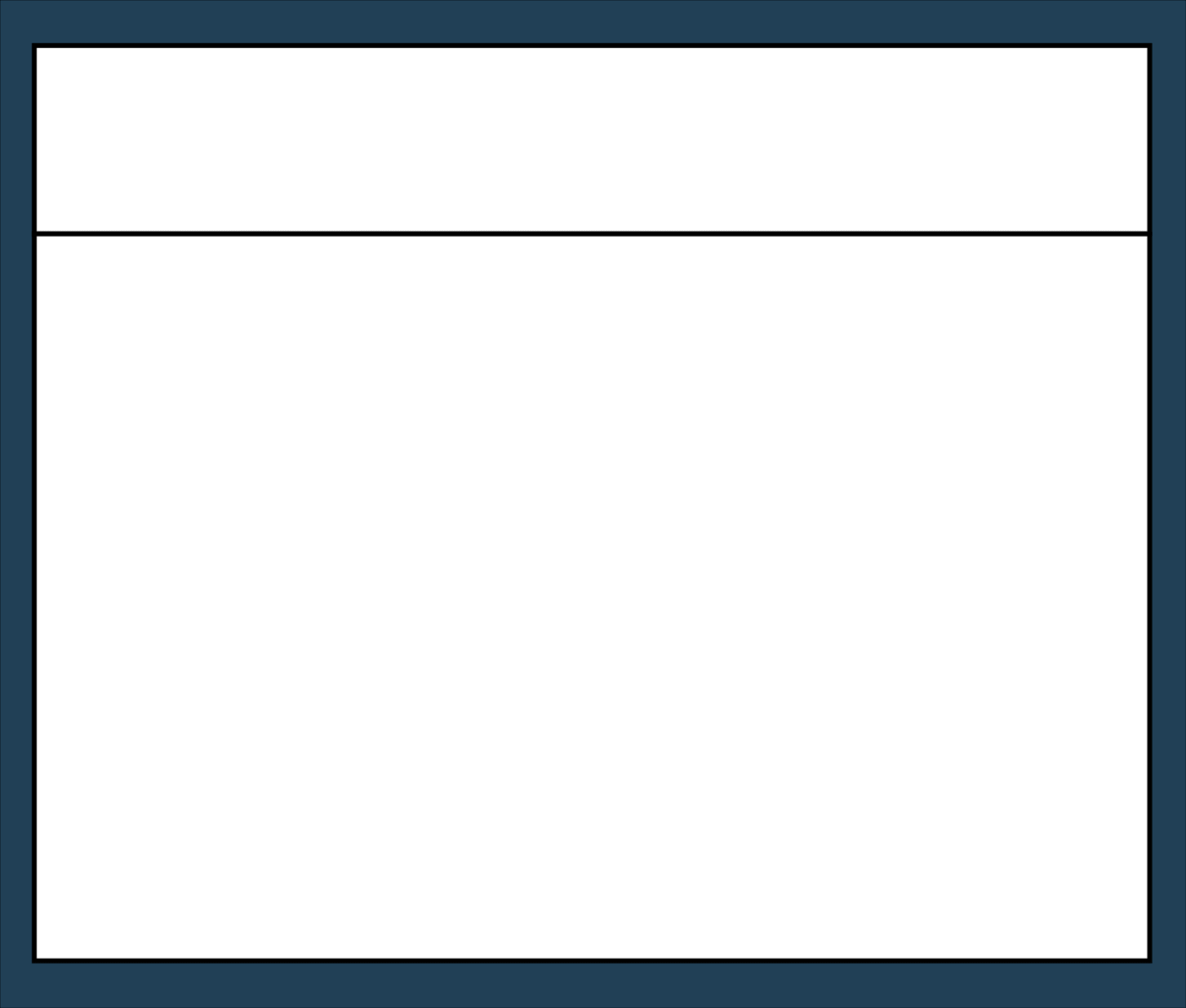In the visual above:

• The first line declares the data that will be used in the plot (`mtcars`) and creates the aesthetic mapping of `wt` to `mpg`.
• The second line creates the data point geom layer.
• The third line creates the smoothed geom layer.

The key is that the `aes()` (aesthetic function) on line one maps the data onto each of the two geom layers

### ggplot() Initializes a ggplot Object

Invoking the `ggplot()` function returns an object that serves as the base of a ggplot2 visualization.

``````viz <- ggplot()
viz # renders blank plot
``````

Data is bound to a ggplot2 visualization by passing a data frame as the first argument in the `ggplot()` function call. Layers can be added to the plot object by adding function calls after `ggplot()` with a `+` plus sign. These functions have access to the data frame and can use the column names as variables.

For example, consider a data frame `sales` with the columns `cost` and `profit`. To assign the data frame `sales` to the `ggplot()` object that is initialized:

``````viz <- ggplot(data=sales) +
geom_point(aes(x=cost, y=profit))
viz # renders plot
``````

In the example above:

• The ggplot object or canvas was initialized with the data frame `sales` assigned to it
• The subsequent `geom_point` layer used the `cost` and `profit` columns to define the scales of the axes for that particular geom. Notice that it referred to those columns with their column names.
• The variable name of the ggplot object is stated so the plot is viewable.

### ggplot2 Aesthetics

In ggplot2 aesthetics are the instructions that determine the visual properties of a plot and its geometries.

Examples of ggplot2 aesthetics include:

• scales for the x and y axes
• color of the data points on the plot based on a property or on a color preference
• the size or shape of different geometries

Aesthetics are set either manually or by aesthetic mappings. Aesthetic mappings “map” variables from the bound data frame to visual properties in the plot. These mappings are provided in two ways using the `aes()` mapping function:

1. At the canvas level: All subsequent layers on the canvas will inherit the aesthetic mappings defined when the ggplot object was created with `ggplot()`.
2. At the geom level: Only that layer will use the aesthetic mappings provided.

For example, the following code assigns `aes()` mappings for the `x` and `y` scales at the canvas level:

``````viz <- ggplot(data=airquality, aes(x=Ozone, y=Temp)) +
geom_point() +
geom_smooth()
``````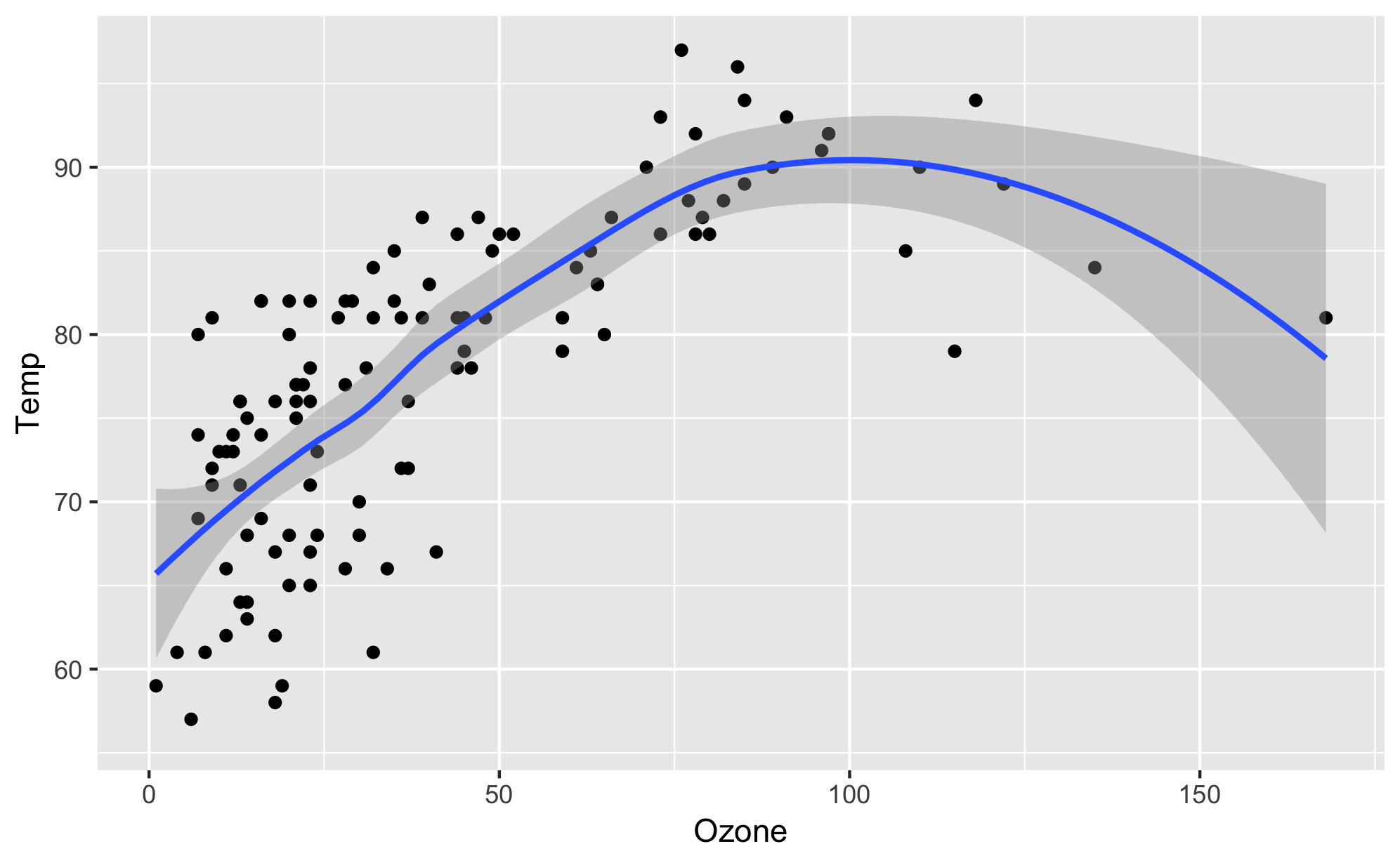In the example above:

• The aesthetic mapping is wrapped in the `aes()` aesthetic mapping function as an additional argument to `ggplot()`.
• Both of the subsequent geom layers, `geom_point()` and `geom_smooth()` use the scales defined inside the aesthetic mapping assigned at the canvas level.

You could create the same plot by setting the aesthetics at the geom level, as follows:

``````viz <- ggplot(data=airquality) +
geom_point(aes(x=Ozone, y=Temp)) +
geom_smooth(aes(x=Ozone, y=Temp))
``````

### Geom Aesthetics

In ggplot2 geom aesthetics are data-driven instructions that determine the visual properties of an individual geom.

Geom aesthetics allow individual layers of a visualization to have their own aesthetic mappings. These aesthetic mappings can vary depending on the geom.

For example, the `geom_point()` geom can color-code the data points on a scatterplot based on a property with the following code:

``````viz <- ggplot(data=airquality, aes(x=Ozone, y=Temp)) +
geom_point(aes(color=Month)) +
geom_smooth()
``````

The code above would only change the color of the point layer, it would not affect the color of the smooth layer since the `aes()` aesthetic mapping is passed at the point layer.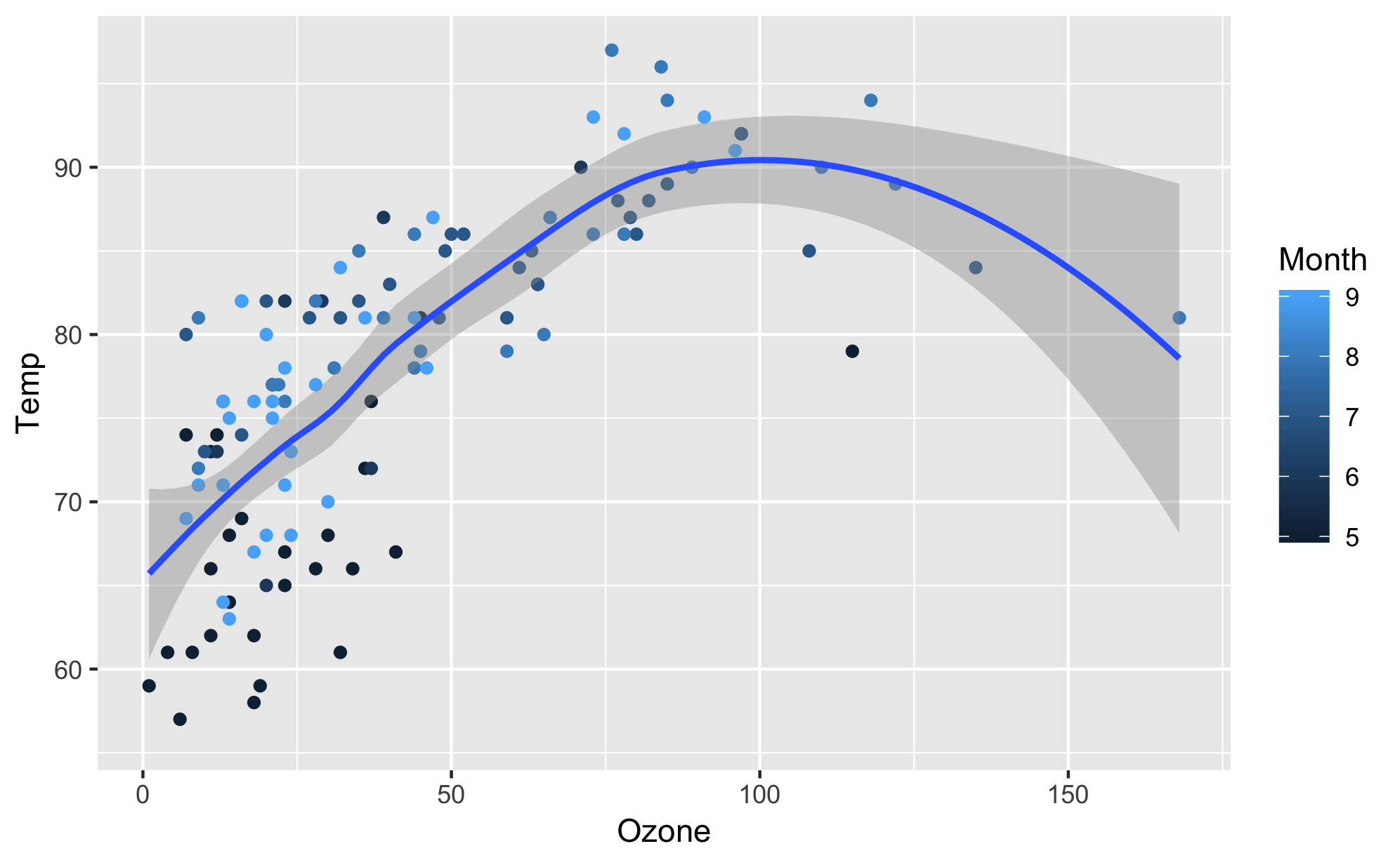### ggplot2 Labels

In ggplot2, labels add meaning and clarity to a data visualization.

ggplot2 automatically assigns the name of the variable corresponding to components, like axes labels. Because data frame variable names are not always legible to outside readers, the `labs()` function allows you to manually set labels.

To customize a plot’s labels, add a `labs()` function call to the ggplot object. Inside the function call to `labs()`, you can provide labels for the `x` and `y` axes as well as a `title`, `subtitle`, or `caption`. The list of available label arguments can be found in the `labs()` documentation.

The following `labs()` function call and these specified arguments would render the following plot:

``````viz <- ggplot(df, aes(x=rent, y=size_sqft)) +
geom_point() +
labs(title="Monthly Rent vs Apartment Size in Brooklyn, NY", subtitle="Data by StreetEasy (2017)", x="Monthly Rent (\$)", y="Apartment Size (sq ft.)")
viz
``````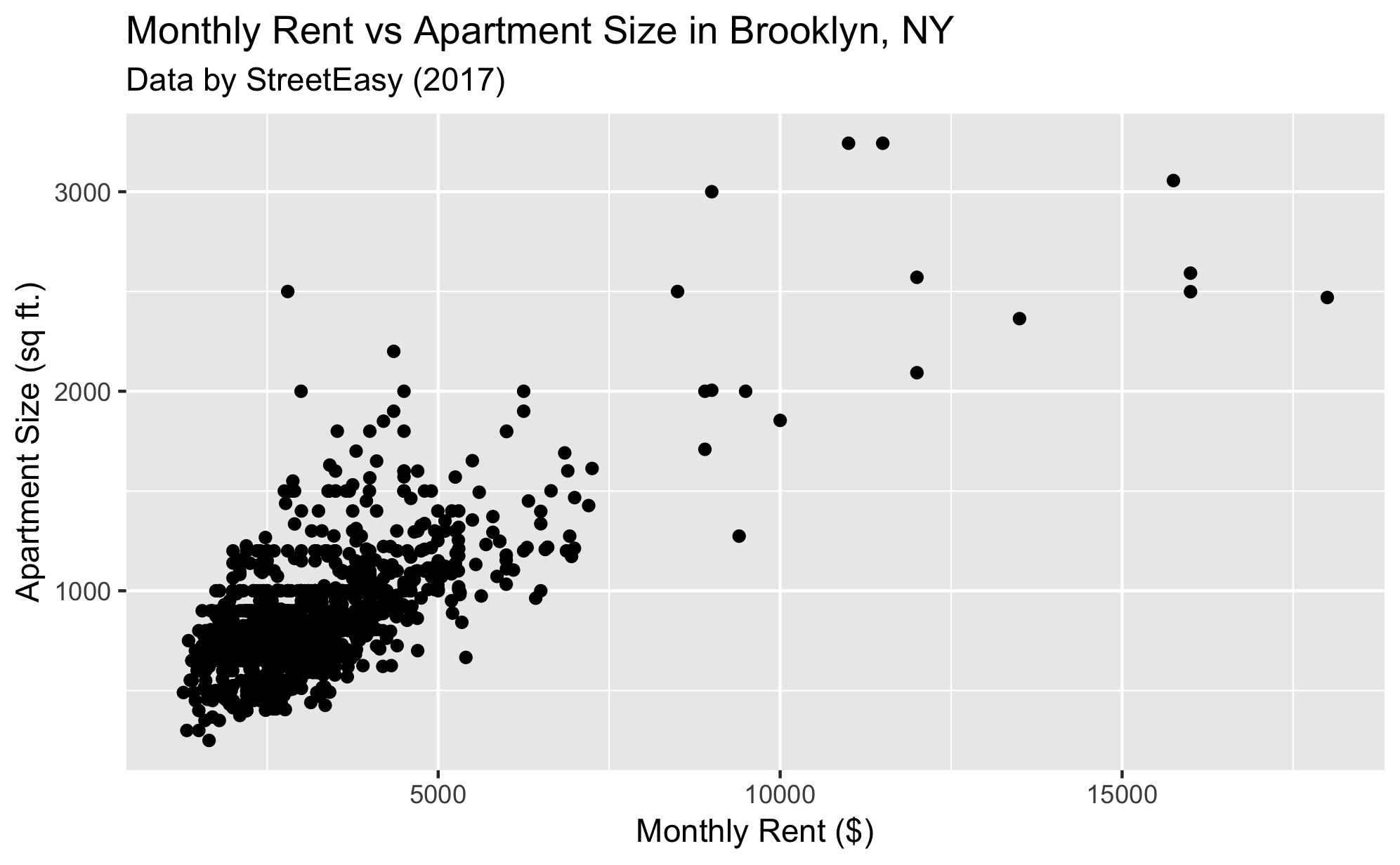### ggplot2 Bar Chart

The `geom_bar()` layer adds a bar chart to a ggplot2 canvas.

Typically when creating a bar chart, an `aes()` aesthetic mapping with a single categorical value on the `x` axes and the `aes()` function will compute the count for each category and display the count values on the `y` axis.

To create a bar chart displaying the number of books in each `Language` from a `books` data frame :

``````bar <- ggplot(books, aes(x=Language)) + geom_bar()
bar
``````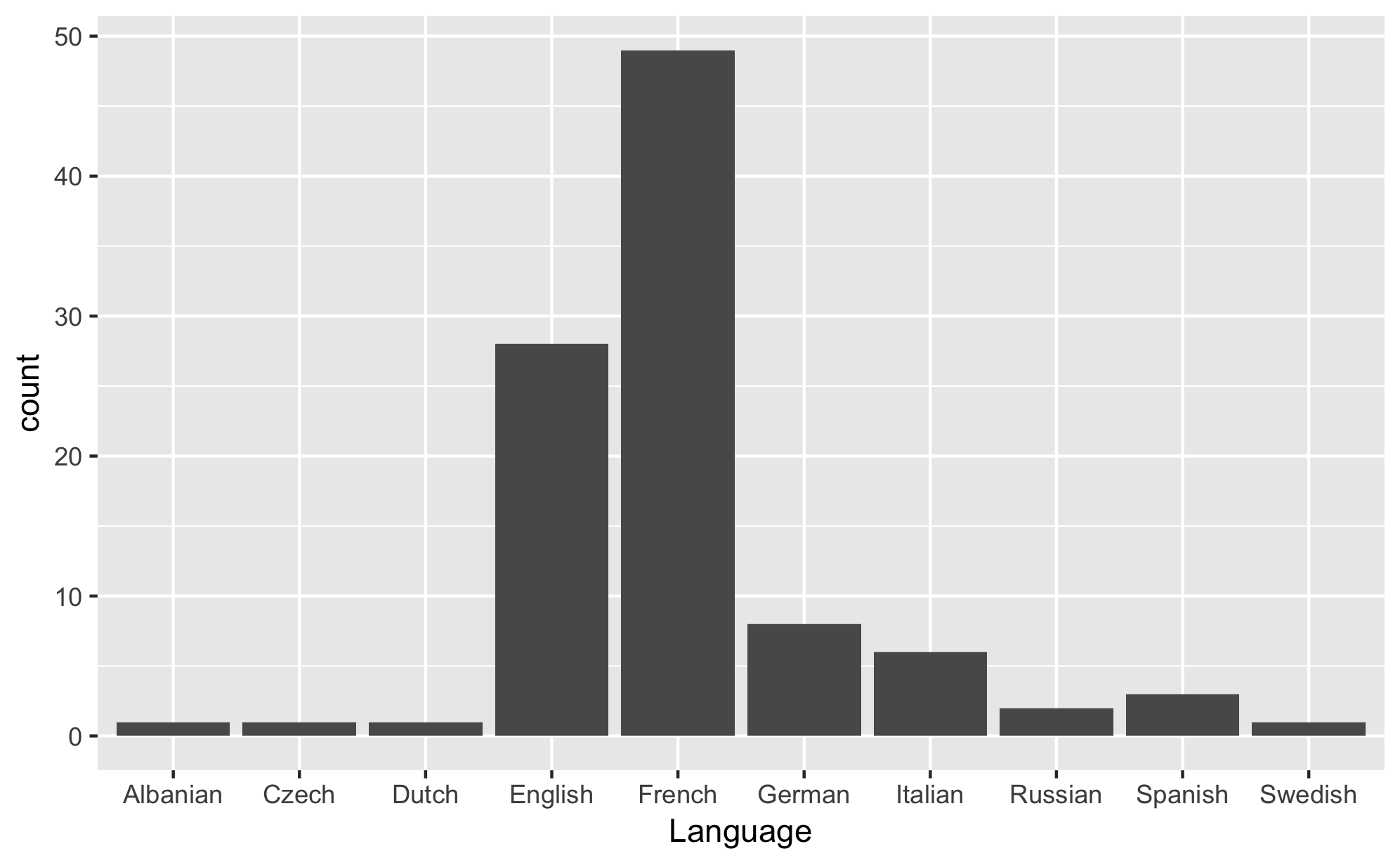Intro to Visualization with R
Lesson 1 of 1
1. 1
Hello there! Today, we will be learning how to make visualizations with R’s popular ggplot2 package. You can create simple visualizations with base R, but we are c…
2. 2
When you learn grammar in school you learn about the basic units to construct a sentence. The basic units in the “grammar of graphics” consist of: + The data or the actual information you wish t…
3. 3
The first thing you’ll need to do to create a ggplot object is invoke the ggplot() function. Conceptualize this step as initializing the “canvas” of the visualization. In this step, it’s also stand…
4. 4
Before we go any further, let’s stop to understand when the data gets bound to the visualization: + Data is bound to a ggplot2 visualization by passing a data frame as the first argument in the gg…
5. 5
In the context of ggplot, aesthetics are the instructions that determine the visual properties of the plot and its geometries. Aesthetics can include things like the scales for the x and y axes, t…
6. 6
Before we teach you how to add aesthetics specific to a geom layer, let’s create our first geom! As mentioned before, geometries or geoms are the shapes that represent our data. In ggplot, there a…
7. 7
In the previous exercises, we added geoms to the plot and explored the idea of layers inheriting the original aesthetic mappings of the canvas. Sometimes, you’ll want individual layers to have thei…
8. 8
We’ve reviewed how to assign data-driven aesthetic mappings at the canvas level and at the geom level. However, sometimes you’ll want to change an aesthetic based on visual preference and not data….
9. 9
So far, we’ve reviewed how to add geometries to represent our data. We’ve also learned how to modify aesthetic values in our plot- whether those aesthetics are data-driven or assigned manually. Ano…
10. 10
We’ve gone over each of the basic units in the grammar of graphics: data, geometries, and aesthetics. Let’s extend this new knowledge to create a new type of plot: [the bar chart](https://ggplot2.t…
11. 11
You’ve completed the introduction to ggplot lesson! You’re ready to follow the general pattern for creating a visualization: 1. Determine what relationship you wish to explore in your data 2. Find…

## What you'll create

Portfolio projects that showcase your new skills## How you'll master it

Stress-test your knowledge with quizzes that help commit syntax to memory